# 神经网络的参数形成与训练

【摘要】 本节主要对于神经网络的参数生成和训练进行讲解，涉及到了前向传播和后向传播的概念与知识！

# 神经网络的参数

## 导言

### 参数形成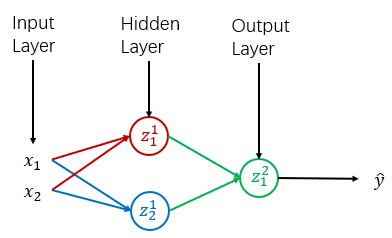``````W = np.random.randn(n_h,n_x)*0.1
b = np.random.zeros((n_h,1))
``````（这时杠精就要来了！）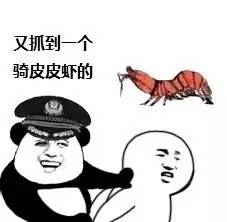### 参数的训练

#### 梯度下降

##### 1. 损失函数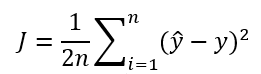``````j = 1/n* np.sum((np.expand_dims(Y,1)-S)**2)
``````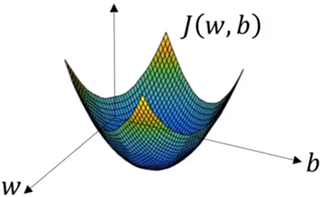##### 2. 求偏导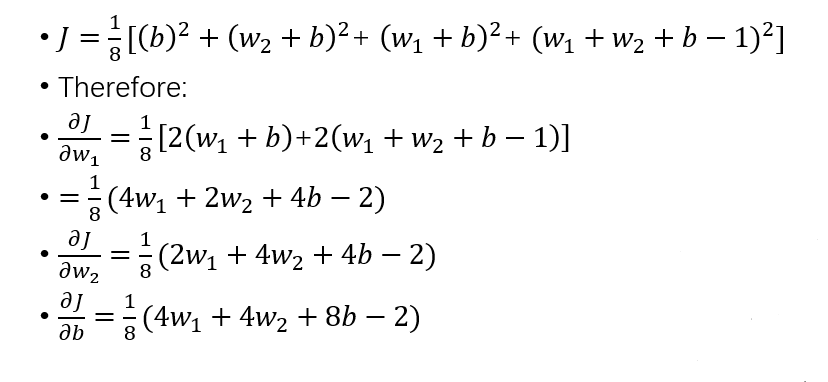##### 3. 更新参数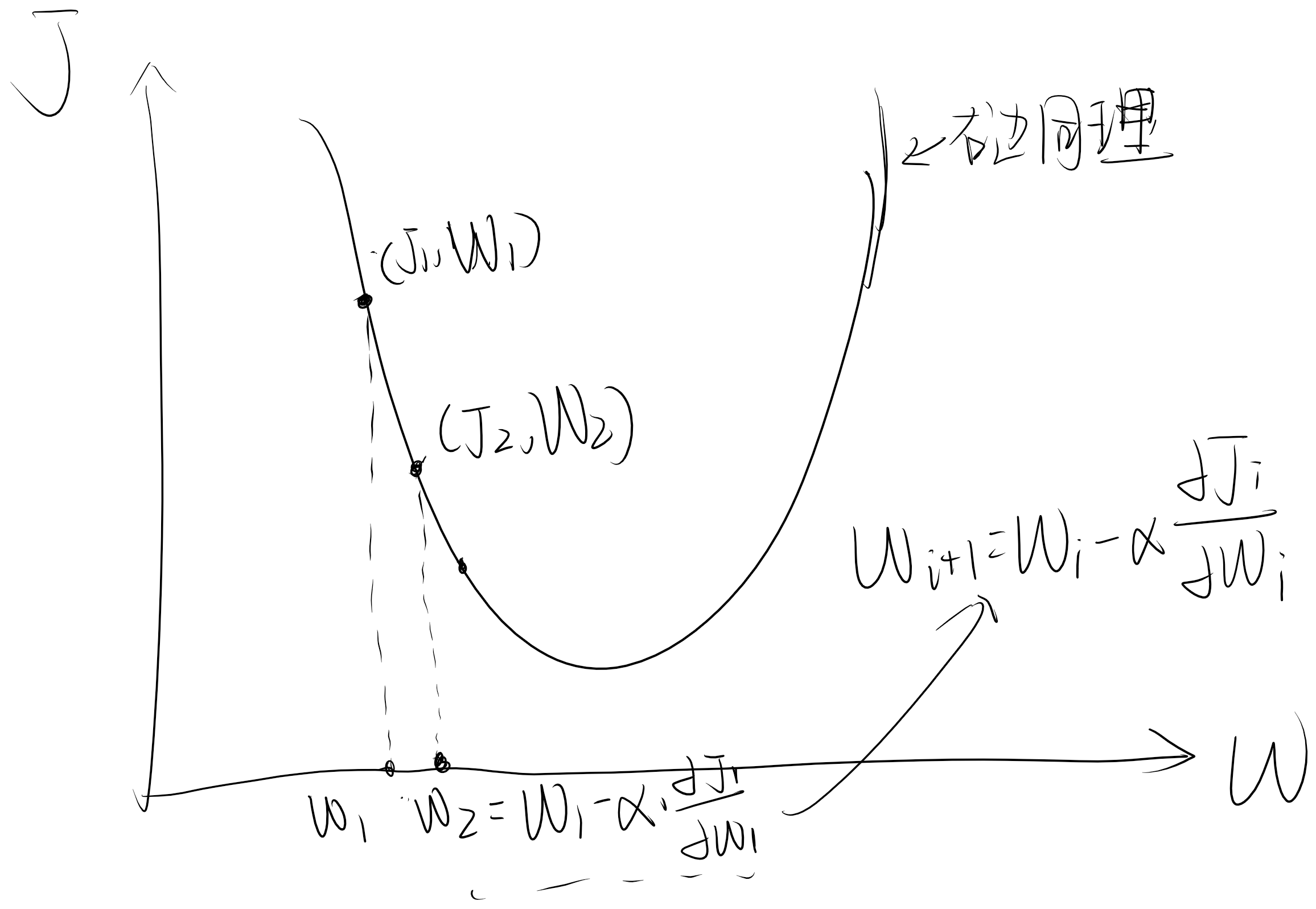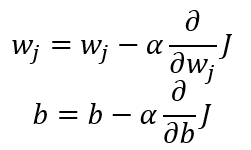``````W = W - α*G
``````### 另一损失函数

（图片）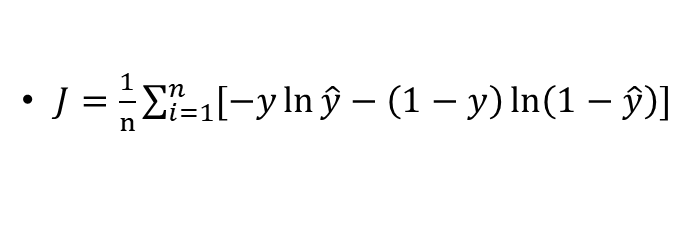``````G = np.dot(X.T, 1 / n * (np.expand_dims(Y, 1) - S) * (-1) * S * (1 - S))
``````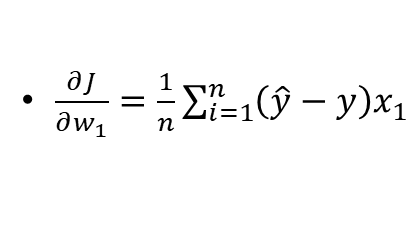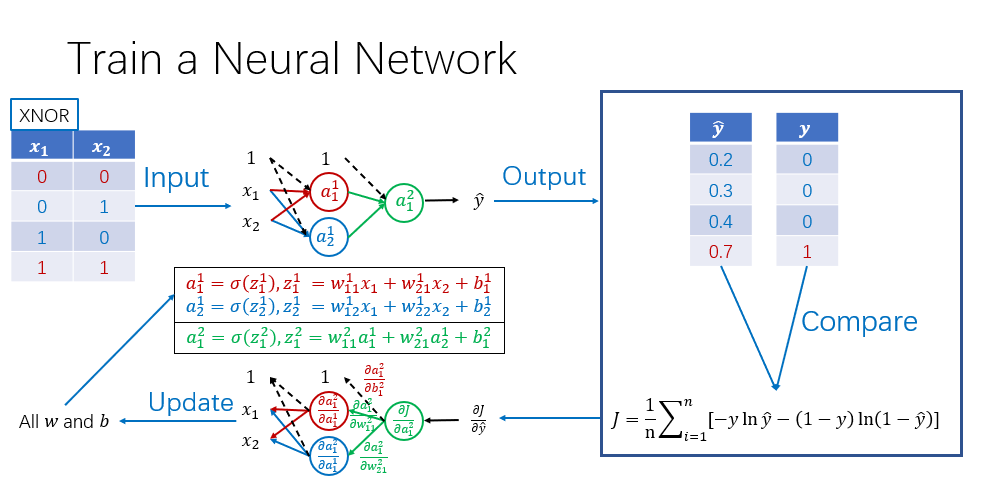## 结言

【版权声明】本文为华为云社区用户原创内容，转载时必须标注文章的来源（华为云社区），文章链接，文章作者等基本信息，否则作者和本社区有权追究责任。如果您发现本社区中有涉嫌抄袭的内容，欢迎发送邮件至：huaweicloud.bbs@huawei.com进行举报，并提供相关证据，一经查实，本社区将立刻删除涉嫌侵权内容。